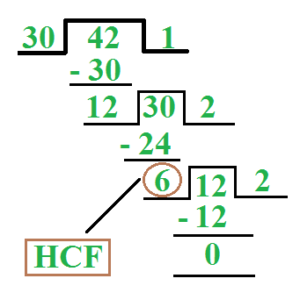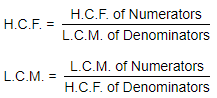# LCM and GCF Calculator

Welcome to hcflcm.com simple math calculator tool. You can calculate GCF known as greatest common factor or greatest common divisor (gcd) and LCM known as least common multiple or lowest common denominator (lcd).

The LCM and GCF calculator (also called the LCD and GCD finder) will determine the least common multiple and greatest common factor of a set of two to n numbers. You can also compute the LCM and GCF by hand or use the LCM calculator or the GCF calculator to find more detailed methods to compute these problems by hand.

## Factors, LCM and GCF of two or more Numbers

Please Select a calculator below to start calculate Factors, LCM (LCD) or GCF (GCD)

### LCM Calculator - LCM of two Numbers

The Least Common Multiple (LCM) is also referred to as the Lowest Common Multiple (LCM) and Least Common Divisor (LCD). For two integers x and y, denoted LCM(x,y), the LCM is the smallest positive integer that is evenly divisible by both x and y.

For example, LCM(2,3) = 6 and LCM(6,10) = 30.

### GCF Calculator - GCF of two Numbers

The Greatest Common Factor (GCF) is also referred to as the Highest Common Factor (HCF) and Greatest Common Divisor (GCD). For two integers x and y, denoted GCF(x,y), the largest positive integer that divides evenly into two numbers with zero remainder.

For example, GCF(12,36) = 12 and GCF(42,64) = 2.

### LCM of two or more Numbers Calculator

The LCM of two or more numbers is the smallest number that is evenly divisible by all numbers in the set.

For example, for the set of numbers 12, 24 and 36 the LCM = 72.

### GCF of two or more Numbers Calculator

The GCF(GCD) of two or more numbers is the largest number that is evenly divisible by all numbers in the set with remainder zero.

For example, for the set of numbers 12, 24 and 36 the GCF = 12.

### HCF Using Euclid’s Division Lemma

Euclid’s division algorithm is a technique to compute the Highest Common Factor (HCF) of two given positive integers. Recall that the HCF of two positive integers a and b is the largest positive integer d that divides both a and b.

For example, HCF (420, 130) = 10.

### Factoring Calculator

The Factoring Calculator finds the factors and factor pairs of a natural number. Enter an positive integer number to find its factors.

For example, The 10 factors of 48 are: 1, 2, 3, 4, 6, 8, 12, 16, 24, 48.

### Prime Factorisation Calculator

Find the prime factorization of a number. Works for natural numbers between 2 and 9007199254740991

Prime Factors of a Number 12 With Exponents = 22 x 3

Prime Factors of a Number 12 Without Exponents = 2 x 2 x 3

### Factor Tree

A factor tree is a tool that breaks down any number into its prime factors. A certain number's prime factorization is the list of prime numbers or prime factors that you would multiply together to create that certain number.

For example, the factors of 32 are 1, 2, 4, 8, 16, 32.

### LCM of Decimals

To find the L.C.M of the given numbers in which decimals are given, first of all we find out the L.C.M of numbers with out decimals. And then we see the number in which the decimal is given in the minimum digits from right to left. We put the decimal in our result which is equal to that number of digits.

For example, the L.C.M of 0.16, 5.4 and 0. 0098 = 2116.8

### GCF of Decimals

To find the G.C.F of the given numbers in which decimals are given, first of all we find out the G.C.F of numbers with out decimals. And then we see the number in which the decimal is given in the minimum digits from right to left. We put the decimal in our result which is equal to that number of digits.

For example, the H.C.F. of 1.20 and 22.5 = 0.30

### LCM of Fractions

Formula to find the LCM of two fractions is:

L.C.M of Fractions = LCM of the numerators/GCF of denominators

For example, 4/5 and 3/7 is 420

### GCF of Fractions

Formula to find the GCF of two fractions is:

G.C.F of Fractions = GCF of the numerators/LCM of denominators

For example, 4/5 and 3/7 is 1/35

### LCM and HCF Formulas, Shortcuts and Tricks with Examples

Factors and Multiples:

• If number p divided another number q exactly, we say that p is a factor of q.
• In this case, q is called a multiple of p.Common Multiple :
A number which is exactly divisible by all the given numbers is “Common multiple”.

Least Common Multiple (LCM) :
The least number which is exactly divisible by all the given numbers is LCM.Common Factor:

A number which divides all the given numbers exactly is “Common factor”.

Highest Common Factor (HCF):

The greatest number that divides all the given numbers exactly is “HCF”.

H.C.F by Method of Prime Factors:

(1) H.C.F or G.C.F of 18 and 24?H.C.F by Method of Division:

(1) H.C.F of 30 and 42?H.C.F and L.C.M of Fractions:Co-primes: Two numbers are said to be co-primes if their H.C.F. is 1.

L.C.M and HCF Important Formulas

• Product of two numbers (First number x Second Number) = H.C.F. X L.C.M.
• H.C.F. of a given number always divides its L.C.M.
• To find the greatest number that will exactly divide x, y and z. Required number = HCF of x, y and z
• To find the Largest number that will divide x, y and z leaving remainders a, b and c respectively. Required number = HCF of (x -a), (y- b) and (z – c)
• To find the least number which is exactly divisible by x, y and z. Required number = LCM of x, y and z
• To find the least number which when divided by x, y and z leaves the remainders a, b and c respectively. It is always observed that, (x – a) = (y – b) = (z- c) = K (say). Required number = (LCM of x, y and z) – K.
• To find the least number which, when divided by x, y and z leaves the same remainder r in each case. Required number = (LCM of x, y and z) + r
• To find the greatest number that will divide x, y and z leaving the same remainder ‘r’ in each case. Required number = HCF of (x -r), (y- r) and (z- r)
• Largest number which divides x, y, z to leave same remainder = H.C.F. of (y-x), (z-y), (z-x).
• HCF of two prime numbers is always 1.
• To find the n-digit greatest number which, when divided by x, y and z,
(i) leaves no remainder (ie exactly divisible)
Following step wise methods are adopted.
Step I: LCM of x, y and z = L
Step II: L) n-digit greatest number (
Remainder (R)
Step III: Required number = n – digit smallest number + (L – R)
(ii) Leaves remainder K in each case
Step I: LCM of x, y and z = L
Step II: L) n-digit greatest number (
Remainder (R)
Step III: Required number = (n-digit greatest number – R) + K
• To find the n – digit smallest number which, when divided by x, y and z.(i) Leaves no remainder (i.e. exactly divisible)
Following steps are followed.
Step I: LCM of x, y and z = L
Step II: LCM) n-digit smallest number (
Remainder (R)
Step III: The required number = n-digit smallest number + (L – R)
(ii) leaves remainder K in each case.
Step I: LCM of x, y and z = L
Step II: LCM) n-digit smallest number (
Remainder (R)
Step III: Required number = n – digit smallest number + (L – R) + K
• To find the least number which on being divided by x, y and z leaves in each case a remiander R, but when divided by N leaves no remainder, following step-wise methods are adopted.
Step I: Find the LCM of x, y and z say (L).
Step II: Required number will be in the form of (LK + R); where K is a positive integer.
Step III: N) L (Quotient (Q)
—–
Remainder (R0)
∴ L = N X Q + R0
Now put the value of L into the expression obtained in step II.
∴ required number will be in the form of (N × Q + R0) K + R
or, (N × Q x K) + (R0K + R)
Clearly N x Q x K is always divisible by N.
Step IV: Now make (R0K + R) divisible by N by putting the least value of K. Say, 1, 2, 3, 4….
Now put the value of K into the expression (LK + R) which will be the required number.
• There are n numbers. If the HCF of each pair is x and the LCM of all the n numbers is y, then the product of n numbers is given by or Product of ‘n’ numbers = (HCF of each pair)n-1 × (LCM of n numbers).

### Frequently Asked Questions on Factors, LCM (LCD) or GCF (GCD or HCF)

What is the difference between LCM and HCF?

LCM stands for Lowest Common Multiple, and HCF stands for Highest Common Factor.

The LCM of two integers is the smallest whole number that appears in both of their times tables, that is, the smallest integer that is a multiple of both numbers.

For example, the LCM of 4 and 6 is 12.

The HCF of two integers is the largest whole number that divides both numbers without leaving a remainder.

For example, the HCF of 16 and 32 is 16.

What is HCF with an example?

HCF or Highest Common Factor of two or more numbers is the greatest factor which divides the numbers. For example, 3 is the HCF of 3 and 6.

What is LCM with an example?

LCM or Least common multiple is the smallest number which is divisible by two or more given numbers. For example, LCM of 2 & 4 is 4.

What is the GCF of 24 and 36?

By prime factorisation, we know; Factors of 36 = 1, 2, 3, 4, 6, 9, 12, 18 and 36; Factors of 24 = 1, 2, 3, 4, 6, 8, 12 and 24; HCF of (24,36) = 12

What is the formula for HCF and LCM?

Product of Two numbers = (HCF of the two numbers) x (LCM of the two numbers)

How can we find the LCM and HCF?

We can find LCM and HCF using prime factorisation and long division method

What is Multiple?

The basic definition of multiple is manifold. In math, the meaning of a multiple is the product result of one number multiplied by another number.

Ex: 7 x 8 = 56.

Here, 56 is a multiple of the integer 7.

What is Factor?

Multiplying two whole numbers gives a product. The numbers that we multiply are the factors of the product.

3 × 5 = 15 therefore, 3 and 5 are the factors of 15.

This also means:

A factor divides a number completely without leaving any remainder.

For example: 30 ÷ 6 = 5, and there is no remainder. So we can say that 5 and 6 are the factors of 30.